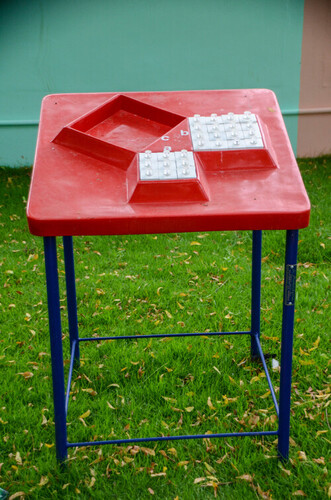# Science Park models Pythagorous TheoremScience Park models Pythagorous Theorem
Product Description

Pythagorous Theorem

Description: Pythagorous theorem states that in any right triangle, the area of the square whose side is the hypotenuse (the side opposite the right angle) is equal to the sum of the areas of the squares whose sides are the two legs (the two sides that meet at a right angle). This theorem explained with these removal cubes.

Recommended Class: 6th, 7th, 8th